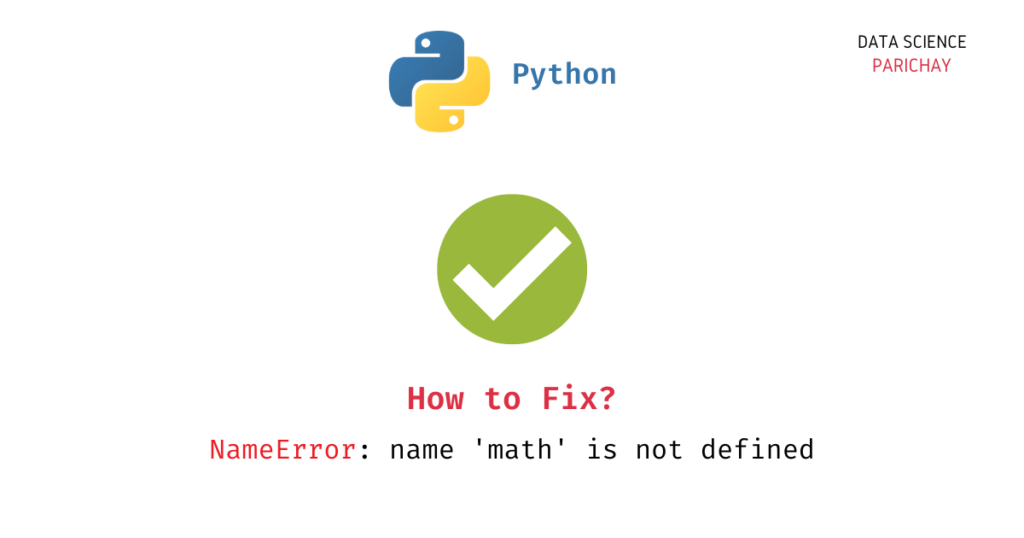# How to Fix – NameError: name ‘math’ is not defined

If you are working with Python and trying to use the math library, you may encounter the “NameError: name ‘math’ is not defined” error. In this tutorial, we will explore why this error occurs and the steps required to fix it such that your Python code can successfully run without errors.

We will cover common causes of the error and provide solutions to help you get your code up and running quickly. So, let’s get started!

## Why does the `NameError: name 'math' is not defined` error occur?

This error occurs when you try to use the math library in your Python code, but Python cannot find the math module in its namespace. The following are some of the scenarios in which this error usually occurs.

1. You have not imported the math module.
2. You have imported the math module using a different name.

## How to fix the `NameError: name 'math' is not defined`?

The `math` library in Python is a built-in module that provides various mathematical operations and functions. It includes functions for basic arithmetic operations, trigonometric functions, logarithmic functions, and more. Since this library is a built-in library in Python, you don’t need to separately install it. You can import it and start using it.

Let’s now look at the above scenarios in detail.

### The `math` module is not imported

It can happen that you are trying to use the `math` module without even importing it. This is because Python does not recognize the math library and its functions until it is imported into the code.

For example, let’s try to use `math` without importing it and see what we get.

```# note that math is not imported

# get the square root of a 4
print(math.sqrt(4))```

Output:

📚 Data Science Programs By Skill Level

Introductory

Intermediate ⭐⭐⭐

🔎 Find Data Science Programs 👨‍💻 111,889 already enrolled

Disclaimer: Data Science Parichay is reader supported. When you purchase a course through a link on this site, we may earn a small commission at no additional cost to you. Earned commissions help support this website and its team of writers.

```---------------------------------------------------------------------------

NameError                                 Traceback (most recent call last)

Cell In, line 4
1 # note that math is not imported
2
3 # get the square root of a 4
----> 4 print(math.sqrt(4))

NameError: name 'math' is not defined```

We get a `NameError` stating that the name `math` is not defined. To use the `math` library, you need to import it first.

```import math

# get the square root of a 4
print(math.sqrt(4))```

Output:

`2.0`

Here, we are importing the `math` module first and then using it to get the square root of 4. You can see that we did not get any errors here.

You can also get a `NameError` if you are importing only specific parts of the library and then trying to access the entire `math` library. For example –

```from math import sqrt

# get the square root of a 4
print(math.sqrt(4))```

Output:

```---------------------------------------------------------------------------

NameError                                 Traceback (most recent call last)

Cell In, line 4
1 from math import sqrt
3 # get the square root of a 4
----> 4 print(math.sqrt(4))

NameError: name 'math' is not defined```

We get a `NameError` here because we are importing only the `sqrt()` function from the `math` library but we are trying to access the entire library. To resolve the above error, either only use the specific method imported or import the `math` library altogether.

### The `math` module is imported using a different name

If you import the math module using a different name, for example `import math as m`, and then try to use the name “math” to use it, you will get a `NameError` because the name “math” is not defined in your current namespace.

Let’s look at an example.

```import math as m

# get the square root of a 4
print(math.sqrt(4))```

Output:

```---------------------------------------------------------------------------

NameError                                 Traceback (most recent call last)

Cell In, line 4
1 import math as m
3 # get the square root of a 4
----> 4 print(math.sqrt(4))

NameError: name 'math' is not defined```

We get a `NameError: name 'math' is not defined`. This is because we have imported the `math` module with the name `m` but we’re trying to use it using the name `math`.

To fix this error, you can either access math using the name that you have used in the import statement or import math without an alias.

```import math as m

# get the square root of a 4
print(m.sqrt(4))```

Output:

`2.0`

In the above example, we are importing `math` as `m` and then using `m` to access the `math` module’s methods.

Alternatively, as seen in the example in the previous section, you can import `math` without any aliases and simply use `math` to avoid the `NameError`.

## Conclusion

In conclusion, encountering a `NameError: name 'math' is not defined` error can be frustrating, but it is a common issue that can be easily fixed. By ensuring that the `math` module is imported correctly and that the correct syntax is used when calling its functions, you can avoid this error and successfully execute your code.

## Author

•Piyush is a data professional passionate about using data to understand things better and make informed decisions. He has experience working as a Data Scientist in the consulting domain and holds an engineering degree from IIT Roorkee. His hobbies include watching cricket, reading, and working on side projects.

Scroll to Top